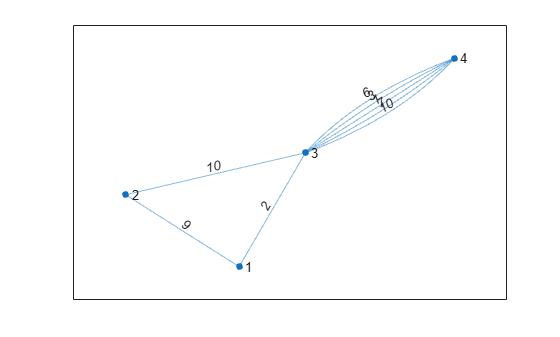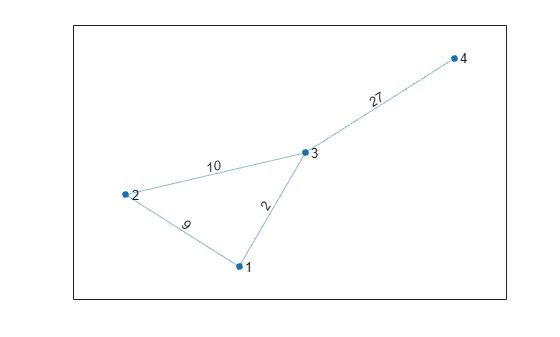# simplify

Reduce multigraph to simple graph

## Syntax

``H = simplify(G)``
``H = simplify(G,pickmethod)``
``H = simplify(G,aggregatemethod)``
``H = simplify(___,selfloopflag)``
``H = simplify(___,Name,Value)``
``[H,eind,ecount] = simplify(___)``

## Description

example

````H = simplify(G)` returns a graph without multiple edges or self-loops. When several edges occur between the same two nodes, only the first edge (as defined in `G.Edges`) is kept. Edge properties are preserved.```

example

````H = simplify(G,pickmethod)` specifies a method to choose between multiple edges. Edge properties are preserved. `pickmethod` can be `'first'` (default), `'last'`, `'min'`, or `'max'`. ```

example

````H = simplify(G,aggregatemethod)` specifies a method to combine the edge weights of multiple edges into the weight of a single new edge. All other edge properties in `G` are dropped. `aggregatemethod` can be `'sum'` or `'mean'`.```

example

````H = simplify(___,selfloopflag)` specifies whether to preserve or remove self-loops from the graph using any of the input argument combinations in previous syntaxes. For example, `'keepselfloops'` specifies that nodes with one or more self-loops will have one self-loop in the simplified graph.```
````H = simplify(___,Name,Value)` specifies additional options with one or more name-value pair arguments. For example, you can specify `'PickVariable'` and a variable in `G.Edges` to use that variable with the `'min'` or `'max'` selection methods.```

example

````[H,eind,ecount] = simplify(___)` additionally returns edge indices `eind` and edge counts `ecount`: `H.Edges(eind(i),:)` is the edge in `H` that represents edge `i` in `G`.`ecount(j)` is the number of edges in `G` that correspond to edge `j` in `H`. ```

## Examples

collapse all

Create a weighted, undirected multigraph with several edges between node 1 and node 2.

```G = graph([1 1 1 1 2 3],[2 2 2 3 3 4], 1:6); G.Edges```
```ans=6×2 table EndNodes Weight ________ ______ 1 2 1 1 2 2 1 2 3 1 3 4 2 3 5 3 4 6 ```

Simplify the multigraph into a simple graph, such that there is only one edge between node 1 and node 2. `simplify` keeps the first edge between those two nodes, `G.Edges(1,:)`, and drops the others.

```G = simplify(G); G.Edges```
```ans=4×2 table EndNodes Weight ________ ______ 1 2 1 1 3 4 2 3 5 3 4 6 ```

Use the second input of `simplify` to select a method that picks between multiple edges or combines multiple edges into one.

Create a weighted multigraph. In this graph, five edges occur between node 3 and node 4, but the edges have random weights. View the edges table and plot the graph for reference.

```G = graph([1 2 3 3 3 3 3 3 ],[2 3 1 4 4 4 4 4],randi(10,1,8)); G.Edges```
```ans=8×2 table EndNodes Weight ________ ______ 1 2 9 1 3 2 2 3 10 3 4 10 3 4 7 3 4 1 3 4 3 3 4 6 ```
`plot(G,'EdgeLabel',G.Edges.Weight)`The command `simplify(G)` keeps the first of the repeated edges. However, you can specify a different pick/combine method with the second input.

The options for picking between multiple edges are: `'first'` (default), `'last'`, `'min'`, and `'max'`. Keep the repeated edge with maximum weight.

```H_pick = simplify(G,'max'); plot(H_pick,'EdgeLabel',H_pick.Edges.Weight)```The options for combining multiple edges into one are: `'sum'` and `'mean'`. Sum repeated edges together to produce a single edge with a larger weight.

```H_comb = simplify(G,'sum'); plot(H_comb,'EdgeLabel',H_comb.Edges.Weight)```Simplify a graph while preserving self-loops using the `'keepselfloops'` option.

Create a multigraph with two nodes and several self-loops. Simplify the graph and preserve self-loops.

```G = graph([1 1 1 1 1 1 1 2 2 2 2],[1 1 1 1 2 2 2 2 2 2 2 ]); plot(G) axis equal``````G = simplify(G,'keepselfloops'); plot(G) axis equal```Use the second and third outputs of `simplify` to get information about how many (and which) edges are combined.

Create an undirected multigraph with three nodes and four edges.

```G = graph([1 1 1 2],[2 2 3 3]); G.Edges```
```ans=4×1 table EndNodes ________ 1 2 1 2 1 3 2 3 ```

Simplify the graph and specify three outputs to get additional information about the combined edges.

`[G,ei,ec] = simplify(G)`
```G = graph with properties: Edges: [3x1 table] Nodes: [3x0 table] ```
```ei = 4×1 1 1 2 3 ```
```ec = 3×1 2 1 1 ```

`ei(i)` is the edge in the simplified graph that represents edge `i` in the old graph. Since the first two edges are repeated, `ei(1) = ei(2) = 1`. Also, `ec(1) = 2`, since there are two edges in the new graph corresponding to edge 1 in the old graph.

Show how to simplify a multigraph using the `'PickVariable'` and `'AggregationVariables'` name-value pairs.

Create a multigraph where the nodes represent locations and the edges represent modes of transport. The edges have properties that reflect the cost and time of each mode of transportation. Preview the edges table.

```G = graph([1 1 1 1 1 1 2 2 2],[2 2 2 3 3 3 3 3 3],[],{'New York', 'Boston', 'Washington D.C.'}); G.Edges.Mode = categorical([1 2 3 1 2 3 1 2 3],[1 2 3],{'Air' 'Train' 'Bus'})'; G.Edges.Cost = [400 80 40 250 100 75 325 150 100]'; G.Edges.Time = [1 7 5 1.5 10 8 1.75 11 9]'; G.Edges```
```ans=9×4 table EndNodes Mode Cost Time ___________________________________ _____ ____ ____ {'New York'} {'Boston' } Air 400 1 {'New York'} {'Boston' } Train 80 7 {'New York'} {'Boston' } Bus 40 5 {'New York'} {'Washington D.C.'} Air 250 1.5 {'New York'} {'Washington D.C.'} Train 100 10 {'New York'} {'Washington D.C.'} Bus 75 8 {'Boston' } {'Washington D.C.'} Air 325 1.75 {'Boston' } {'Washington D.C.'} Train 150 11 {'Boston' } {'Washington D.C.'} Bus 100 9 ```

Plot the graph for reference. Label the transportation mode on each edge, make the edge line widths proportional to the time, and the color of each edge proportional to the cost.

```plot(G,'EdgeLabel',cellstr(G.Edges.Mode),'LineWidth',G.Edges.Time./min(G.Edges.Time),'EdgeCData',G.Edges.Cost) colorbar```Use the `'min'` selection method and specify the value of `'PickVariable'` as the `'Time'` variable to find the quickest mode of transport between each set of nodes.

```t = simplify(G,'min','PickVariable','Time'); plot(t,'EdgeLabel',cellstr(t.Edges.Mode))```Use the `'sum'` aggregation method and specify the value of `'AggregationVariables'` as `'Cost'` to compute how much money is made on each connection.

```c = simplify(G,'sum','AggregationVariables','Cost'); plot(c,'EdgeLabel',c.Edges.Cost)```## Input Arguments

collapse all

Input graph, specified as either a `graph` or `digraph` object. Use `graph` to create an undirected graph or `digraph` to create a directed graph.

Example: `G = graph(1,2)`

Example: `G = digraph([1 2],[2 3])`

Edge picking method, specified as `'first'`, `'last'`, `'min'`, or `'max'`. The edge picking method provides a way to choose which of several edges to preserve when more than one edge exists between the same two nodes.

• If the method is `'first'` or `'last'`, then `simplify` preserves only the first or last edge that occurs in the edges table `G.Edges`.

• If the method is `'min'` or `'max'`, then `simplify` preserves only the edge with minimum or maximum weight. The `Weight` variable must exist in `G.Edges`, unless you use the `'PickVariable'` name-value pair to base the selection on a different variable.

Example: `simplify(G,'last')`

Aggregation method, specified as either `'sum'` or `'mean'`. The aggregation method provides a way to combine several edges into a single edge when there is more than one edge between the same two nodes.

By default, `simplify` only sums or averages the edge weights in the graph and drops all other edge properties. However, you can use the `'AggregationVariables'` name-value pair to specify which numeric variables in `G.Edges` to preserve and aggregate.

Example: `simplify(G,'sum')`

Toggle to keep self-loops, specified as either:

• `'omitselfloops'` — Remove all self-loops from the graph. This is the default.

• `'keepselfloops'` — Nodes with one or more self-loops have a single self-loop in the simplified graph.

Example: `simplify(G,'sum','keepselfloops')`

### Name-Value Pair Arguments

Specify optional comma-separated pairs of `Name,Value` arguments. `Name` is the argument name and `Value` is the corresponding value. `Name` must appear inside quotes. You can specify several name and value pair arguments in any order as `Name1,Value1,...,NameN,ValueN`.

Example: ```H = simplify(G,'sum','AggregationVariables',{'Var1' 'Var2'})```

Variable to base edge selection on, specified as the comma-separated pair consisting of `'PickVariable'` and a variable name or numeric index. Use this name-value pair to select an edge variable in `G.Edges` other than `'Weight'` to use with the `'min'` or `'max'` picking methods. `simplify` preserves only the edge with the minimum or maximum value of the selected variable when there are several edges between the same two nodes.

Example: `simplify(G,'min','PickVariable',3)`

Example: `simplify(G,'min','PickVariable','var3')`

Data Types: `single` | `double` | `int8` | `int16` | `int32` | `int64` | `uint8` | `uint16` | `uint32` | `uint64` | `char` | `string`

Variables to aggregate, specified as the comma-separated pair consisting of `'AggregationVariables'` and a variable name, cell array of variable names, string array, numeric vector, logical vector, or function handle. Use this name-value pair to select one or more edge variables in `G.Edges` that can be combined with the `'sum'` or `'mean'` aggregation methods. `simplify` combines the values of these variables into a single value for one new edge when there are several edges between the same two nodes. The value of `'AggregationVariables'` can be one of the following:

• Character vector specifying a single table variable name

• Cell array of character vectors where each element is a table variable name

• String array specifying one or more variable names

• Vector of table variable indices

• Logical vector whose elements correspond to table variables, where `true` includes the corresponding variable and `false` excludes it

• A function handle that takes the `G.Edges` table as input and returns a logical scalar, such as `@isnumeric`

Example: ```simplify(G,'sum','AggregationVariables',[4 5 6])```

Example: ```simplify(G,'mean','AggregationVariables',{'var5 var7'})```

Example: `simplify(G,'mean','AggregationVariables',@isnumeric)`

Data Types: `single` | `double` | `logical` | `function_handle` | `char` | `string` | `cell`

## Output Arguments

collapse all

Simplified graph, returned as a `graph` or `digraph` object. `H` does not contain any repeated edges between the same two nodes, such that `ismultigraph(H)` returns logical `0` (`false`). Self-loops also are removed, unless you specify the `'keepselfloops'` option.

Edge indices, returned as a vector. The edge in `H` that represents edge `i` in `G` is given by `H.Edges(eind(i),:)`. If edge `i` in `G` is a self-loop that was removed, then `eind(i)` is `0`.

Edge counts, returned as a vector. `ecount(i)` is the number of edges in `G` that correspond to edge `i` in `H`.